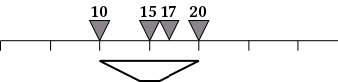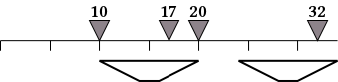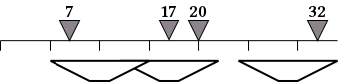## Dancing Elephants

Dancing Elephants is a spectacular show in Pattaya that features N elephants dancing on a line, known as the stage.

After years of training, elephants in this show are capable of many amazing dances. The show consists of a series of acts. In each act, exactly one elephant performs a cute dance while possibly moving to a different position.

The show producers want to produce a photo book that contains pictures of the entire show. After each act, they want to take pictures of all elephants as seen by the spectators.

At any time during the show, multiple elephants may share the same position. In that case, they simply stand behind one another at the same position.

A single camera can take a picture of a group of elephants if and only if all their positions lie on some segment of length L (including both its endpoints). As the elephants can spread out across the stage, multiple cameras may be needed in order to take simultaneous snapshots of all the elephants.

As an example, suppose that L=10 and that the elephants are at positions 10, 15, 17, and 20 on the stage. At this moment, a single camera can take their picture, as shown below. (Elephants are shown as triangles; cameras are shown as trapezoids.)In the following act, the elephant at position 15 dances to position 32. After this act, we need at least two cameras to take the snapshot.In the next act, the elephant at position 10 moves to position 7. For the new arrangement of elephants, we need three cameras to photograph all of them.In this interactive task, you have to determine the minimum number of cameras needed to take the pictures after each of the acts. Note that the number of cameras needed may increase, decrease, or stay the same between acts.

Write a program that takes the following parameters at first:

• N — the number of elephants. The elephants are numbered 0 through N-1.
• L — the length of the segment captured by a single camera. You may assume that L is an integer such that 0 ≤ L ≤ 1 000 000 000.
• M — the number of update calls (see below).
• X — a one-dimensional array of integers representing the initial positions of the elephants. For 0 ≤ i < N, elephant i starts at the position X[i]. The initial positions are in sorted order. More precisely, you may assume that 0 ≤ X ≤ … ≤ X[N-1] ≤ 1 000 000 000. Note that during the dance the elephants may reorder themselves.

Then, there will be M update calls, each consisting of the following parameters:

• i — the number of the elephant that moves in the current act.
• y — the position where the elephant i will stand after the current act. You may assume that y is an integer such that 0 ≤ y ≤ 1 000 000 000.

Each call corresponds to a single act (which follows on from all of the previous acts). For each call, your program must output the minimum number of cameras needed to photograph all elephants after the corresponding act.

### Examples

Consider the case where N=4, L=10, M=5, and the initial positions of the elephants are

 X= ```10 15 17 20```

The input will first consist of the above parameters. Afterwards, there will be M update calls. Here is an example sequence of calls and their correct output values:

actcall parametersoutput value
1update(2,16)1
2update(1,25)2
3update(3,35)2
4update(0,38)2
5update(2,0)3

### Input Format

• Line 1: N, L, and M
• Lines 2 to N+1: the initial positions; i.e., line k+2 contains X[k] for 0 ≤ k < N.
• Lines N+2 to N+M+1: information on M acts; i.e. line N+1+j contains i[j], y[j], and s[j], separated by a space, denoting that in the jth act elephant i[j] moves to position y[j], for 1 ≤ j ≤ M.

### Output Format

• Lines 1 to M: s[j], the minimal number of cameras needed after the elephant moved in the jth act for 1 ≤ j ≤ M

### Sample Input

```4 10 5
10
15
17
20
2 16
1 25
3 35
0 38
2 0```

### Sample Output

```1
2
2
2
3```

#### Subtask 1 (10% of points)

• There are exactly N = 2 elephants.
• 1 ≤ M ≤ 100.
• Initially, and after each act, the positions of all elephants will be distinct.

#### Subtask 2 (16% of points)

• 1 ≤ N ≤ 100.
• 1 ≤ M ≤ 100.
• Initially, and after each act, the positions of all elephants will be distinct.

#### Subtask 3 (24% of points)

• 1 ≤ N50 000.
• 1 ≤ M50 000.
• Initially, and after each act, the positions of all elephants will be distinct.

#### Subtask 4 (47% of points)

• 1 ≤ N70 000.
• 1 ≤ M70 000.
• Elephants may share the same position.

#### Subtask 5 (3% of points)

• 1 ≤ N150 000.
• 1 ≤ M150 000.
• Elephants may share the same position.
• Note: The collection templates in the C++ Standard Library (STL) can be slow; in particular, it might not be possible to solve this subtask if you use them.

Point Value: 30 (partial)
Time Limit: 10.00s
Memory Limit: 256M